# How to Choose the Right Inductor for DC-DC Buck Applications

DC-DC converters of different varieties are widely used in markets such as telecommunications, automotive, medical (portable), and industrial. The ‘buck’ DC-DC converter is employed to step voltages down without isolation and utilizes an inductor as an energy storage element. This article will explain how to choose the correct inductor for optimum performance.

A simplified buck converter schematic can be observed in Figure 1. The output voltage of this converter is always lower than the input voltage.

When S1 is open, the energy stored in the inductor sustains the current through the load through D1 with C1 also discharging into the load. S1 switches at high frequency and the duty cycle of switching defines the output voltage.

When S1 is closed, the DC input voltage is applied to the output filter inductor L1 and current flows through the inductor into the output capacitor C1 and to the load.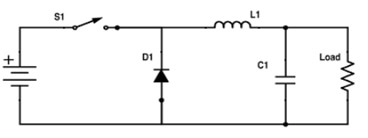Figure 1. Simplified buck schematic

When selecting an inductor for a buck converter the following parameters need to be defined:

• Maximum input voltage = Vin max
• Minimum input voltage = Vin min
• Maximum output current = Iout max
• Operating frequency = f
• Output voltage = Vout
• Minimum output current = Iout min

So, for example, with f = 500 kHz, Vo = 5 volts, Iout max = 4 amps, Iout min = 0.5 amps, Vin max = 13.5 volts (car battery) and Vin min = 8.5 volts.

You can select what the inductor ripple current is – it sets output ripple voltage across the ESR of C1.

For this example, the inductor current stays continuous at the minimum specified load. So, the minimum of the ripple current just touches zero at minimum load, as seen in Figure 2. This makes control loop compensation a lot simpler. The inductor current stays continuous at any load, even with high ripple current, if D1 is a synchronous rectifier MOSFET. Though high ripple current does generate higher core losses.

## Calculations

Since the operating frequency is 500 kHz,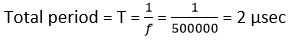Find the minimum duty ratio, Dmin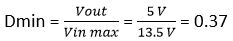Find the required inductance value, L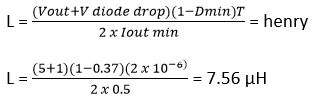Find the delta current (ripple current), ∆I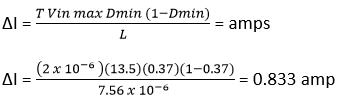Find the peak current, I pk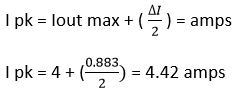Find the RMS current, I rms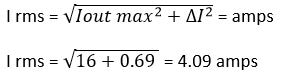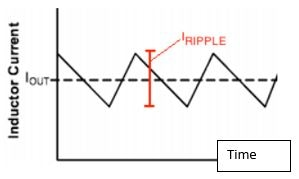Figure 2. Typical inductor ripple current

An optimum inductor can be chosen using TT Electronics (TT) inductor datasheets. In this example, TT part number HA72L-06308R2LFTR is suggested, which is automotive grade, -55 ºC to +155 ºC (AEC-Q200 certified) with the electrical parameters below:

Table 1. Data sheet specification for TT part HA72L-06308R2LFTR

TT p/n L @ 0 amp DCR typical DCR max I rms I dc (sat) 30% Roll off Typical picture
HA72L-06308R2LFTR 8.2 µH 64 mΩ 68 mΩ 4.0 amps gives about 40 ºC Temp Rise 7.5 amps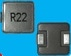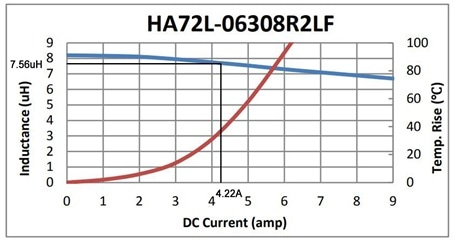Figure 3. HA72L-06308R2LF DC bias and Temp rise

Figure 3 shows the inductance roll-off is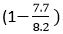100 = 6.1% at 4.42 amps DC, which is close to the required value of 7.56 µH with a temperature rise of less than 40 °C.

The temperature rise in the data sheet graph is for DC current. In the example, there is also AC ripple current. A more precise temperature rise calculation, including ohmic and AC loss effects in the core and wire, is beyond the scope of this article but would be dependent on the below parameters:

1. Skin depth at the operating frequency
2. Surface area of the inductor
3. Wire resistance at the operating temperature
4. AC flux density, BAC, calculated from peak current, inductance, core cross sectional area and number of turns

TT Electronics has provided numerous solutions to the medical, automotive, and various industrial sectors. They provide semi and full custom designs to meet your critical specifications.This information has been sourced, reviewed and adapted from materials provided by TT Electronics.

## Citations

• APA

TT Electronics plc. (2019, October 29). How to Choose the Right Inductor for DC-DC Buck Applications. AZoSensors. Retrieved on June 04, 2020 from https://www.azosensors.com/article.aspx?ArticleID=1644.

• MLA

TT Electronics plc. "How to Choose the Right Inductor for DC-DC Buck Applications". AZoSensors. 04 June 2020. <https://www.azosensors.com/article.aspx?ArticleID=1644>.

• Chicago

TT Electronics plc. "How to Choose the Right Inductor for DC-DC Buck Applications". AZoSensors. https://www.azosensors.com/article.aspx?ArticleID=1644. (accessed June 04, 2020).

• Harvard

TT Electronics plc. 2019. How to Choose the Right Inductor for DC-DC Buck Applications. AZoSensors, viewed 04 June 2020, https://www.azosensors.com/article.aspx?ArticleID=1644.

## Tell Us What You Think